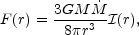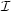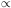### 2. EARLY ACCRETION DISK MODELS

Even if one assumes that angular momentum plays an important role in the dynamics of the central accretion flow, the flow geometry is still very uncertain. The simplest assumption that one can make is that the radial dynamics of the flow is dominated by gravitation and rotation, with radiation pressure, gas pressure, and magnetic forces being negligible by comparison. The plasma in the disk then orbits the black hole on nearly circular test particle ("Keplerian") orbits, and the flow takes the form of a geometrically thin disk. The vertical extent of this disk is determined by balancing the small (by assumption!) internal pressure of the disk with the small vertical component of gravity from the central black hole. At large radii, self-gravity can also become important. The thin disk assumption necessarily breaks down at high accretion luminosities approaching the Eddington limit, where radiation pressure becomes important. In addition it may not be a good assumption at very low accretion rates. We will discuss these complications further in Section 5 below.

The fundamental uncertainty that plagues all theoretical models of accretion disks is the mechanism of outward angular momentum transfer, which is responsible for the slow inward spiral of material that converts gravitational potential energy into radiation and/or an outflow. There are two particularly promising mechanisms for angular momentum transfer in disks, both of which involve magnetic fields. The first is a magnetohydrodynamic (MHD) outflow, where magnetic fields supply torques to the disk and carry away angular momentum in the outflow (Blandford & Payne 1982). The second is internal MHD turbulence, as seen in simulations of the nonlinear development of the Balbus-Hawley instability (Balbus & Hawley 1991; Stone et al. 1996; Balbus & Hawley 1998). Very few models of the radiation produced in the accretion flow are based on these ideas, however, and generally angular momentum transport is treated through a parameterized viscous stress. The most popular prescription is the-viscosity of Shakura & Sunyaev (1973), in which the radial/azimuthal component of the viscous stress is taken to be a parametertimes the total (gas plus radiation) pressure. We will have very little to say here about the detailed mechanisms whereby gravitational energy is converted to radiative and kinetic power in AGNs. Nevertheless, until this is understood, models and interpretation of observations will be severely handicapped.

The basic theoretical models of stationary, geometrically thin disks around black holes were developed by Shakura & Sunyaev (1973) and Novikov & Thorne (1973) (see also Page & Thorne 1974; Eardley & Lightman 1975; Riffert & Herold 1995; and Abramowicz, Lanza, & Percival 1997 for important corrections and refinements to these fundamental papers). From the point of view of predicting the observed spectrum, such models are the most well constrained of all the possible accretion flows considered to date. They make several key assumptions about the flow. In particular, the disk is stationary and axisymmetric and extends down to the innermost stable circular orbit, where zero stress is assumed to be exerted on the disk. Inside this point material falls quickly into the hole and emits very little radiation. Angular momentum transport within the disk occurs by local "viscous" stresses that convert gravitational energy entirely into heat. Because the disk is thin, this heat is assumed to flow vertically out of the disk, thereby being emitted at the same radius as it was generated. Under these assumptions, the radiative flux emerging from each face of the disk at radius r is given by(1)

where M is the mass of the black hole,is the mass accretion rate, and(r) is a correction factor that depends on the mass and angular momentum of the hole and approaches unity at large radii. The local disk flux therefore depends only on the properties of the black hole and the accretion rate. At large radii, F(r)r-3, which implies that the effective temperature of the disk surface varies as Teffr-3/4.

The geometry of the thin disk allows one to calculate the overall emergent spectrum from this total flux by dividing the disk into concentric annuli, calculating the spectrum emitted by each annulus and then summing them all together. The very simplest assumption is that each annulus radiates like a blackbody. The r-3/4 effective temperature distribution at large radii then gives a long wavelength SED of F1/3. As we discuss in Section 3.1 below, this is much bluer than is typically observed in the optical/UV spectra of AGNs.

The earliest attempt at calculating the detailed overall spectrum of optically thick, geometrically thin accretion disks using stellar atmosphere models appropriate for AGNs was by Kolykhalov & Sunyaev (1984). They used the-viscosity prescription to calculate the vertical structure of each annulus. They then took existing stellar atmosphere models from the libraries existing at that time (e.g., Kurucz 1979) with appropriate surface gravities and effective temperatures and then summed them together to generate overall spectra. The most interesting result of their calculations was the existence of very large absorption edges at the hydrogen Lyman limit - edges that existed even when the smearing effects of the relativistic transfer function were included. As we discuss in Section 3.2 below, such edges are not observed, and they noted this discrepancy at the time. This problem motivated much of the later theoretical and observational work on the Lyman edge region of the spectra of AGNs. But as we discuss in Section 4, these early calculations suffered from severe limitations.
Because electron-scattering opacity is important in the disk atmosphere at the expected temperatures and densities, the radiation emerging from the disk can be substantially polarized. Most of the early work assumed that the polarization would be similar to that emerging from an optically thick, pure electron-scattering atmosphere. In this case the polarization is perpendicular to the disk axis and has a strong aspect angle dependence, varying from 0% for a face-on disk (because of symmetry) to 11.7% for an edge-on disk (Chandrasekhar 1960; Rees 1975; Lightman & Shapiro 1975). Pioneering work in the 1980s showed that optical polarization in AGNs is generally much lower (1%) than predicted by these simple models. Often no polarization is detected, and the polarizations are parallel to the nuclear axes, when the latter can be inferred from a radio jet position angle (Stockman, Angel, & Miley 1979; Antonucci 1988).